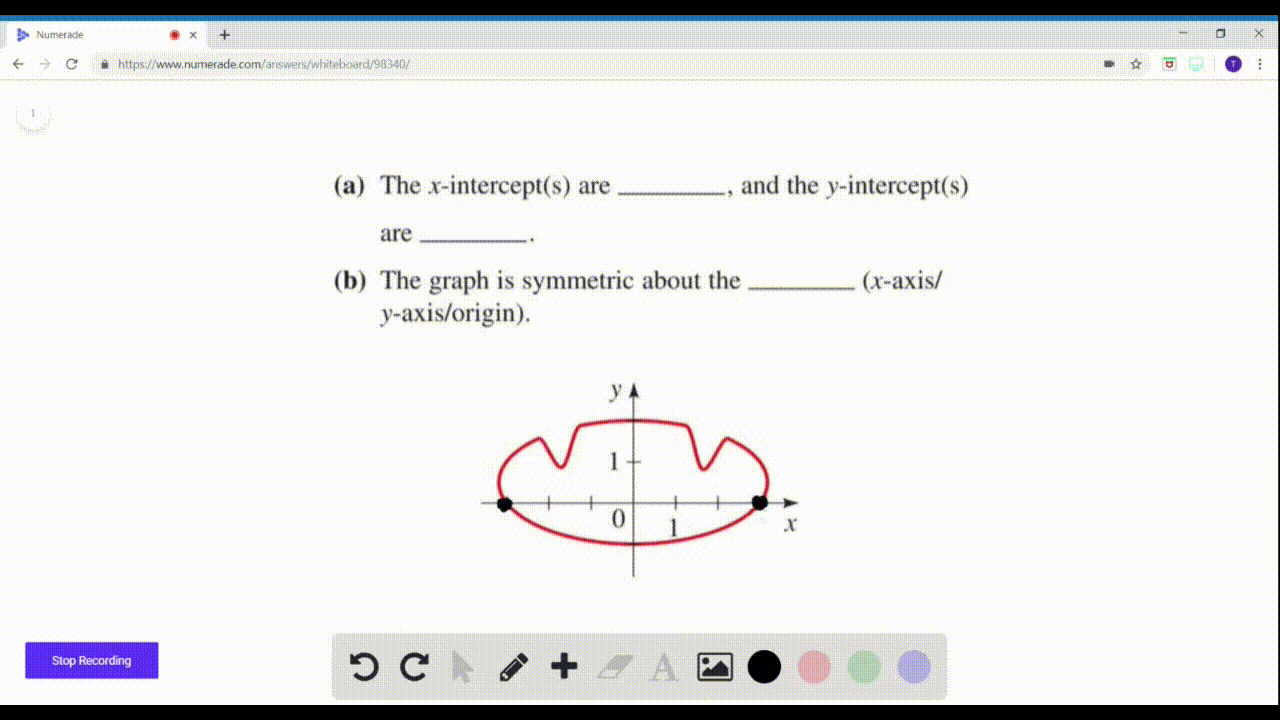🎉 The Study-to-Win Winning Ticket number has been announced! Go to your Tickets dashboard to see if you won! 🎉View Winning Ticket### The graph of an equation is shown below. (a) The …

02:06Problem 5

# (a) If a graph is symmetric with respect to the $x$ -axis and $(a, b)$ is on the graph, then (_____, _____) is also on the graph.(b) If a graph is symmetric with respect to the $y$ -axis and $(a, b)$ is on the graph, then (_____, _____) is also on the graph.(c) If a graph is symmetric about the origin and $(a, b)$ is on the graph, then (_____, _____) is also on the graph.

## Discussion

You must be signed in to discuss.

## Video Transcript

this particular question, I asked you three questions about the symmetry of functions. The first, with respect to the attacks, is a second with respect to the Y axis in the third. With respect to the origin, they all start off with the abstract point to a B, both of them positive. We're going to take those values. This is the strategy can use often. And we're just gonna make those values concrete for now to help us with our examination So we can discover this answer you. We've got a 0.23 on our coordinate plane and we're wondering is what other point would be on this grid if it were symmetric with respect to the X axis. So here symmetry with respect to the X axis would mean that we would have this mirror image across the X axis. So of course, this would be the other point Noticed. The X coordinate doesn't change, but the why does so the answer here, then, for a b also a negative B would be on the graph. No, let's consider the y Axis and let's again use to three. And this time we're going to mirror that point across the Y axis, so we would come here. This point now would be negative, too. But notice that the Y coordinate doesn't change. So here not only would a bee beyond the graph, but so would negative, eh? Comma be and then they hear. Let's consider symmetry with respect to the origin. And again, let's work with that 0.23 This time around, you could consider it across the origin. Or you could consider a double symmetry, a reflection across the X axis and then over the Y or vice versa. But in any case, this 23 becomes negative, too Negative. Three. So going back to the original prompt which posed a comma be we would say that native, a native be is on the same graph.